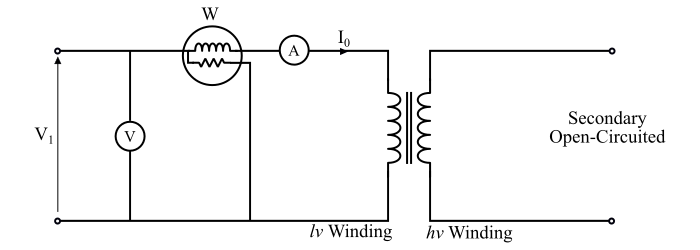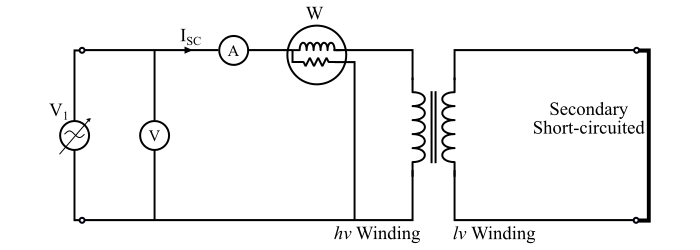# Open Circuit and Short Circuit Test of Transformer

The open-circuit test and the short-circuit test being performed on a transformer to determine the circuit parameters, efficiency and the voltage regulation without actual loaded of the transformer. The open-circuit and short-circuit tests give more accurate results than those are obtained by performing the measurements on a fully loaded transformer. The principle of advantage of these tests is the power consumption is very small as compared to the full-load output of the transformer.

## Open-Circuit Test of Transformer

The circuit arrangement for the open-circuit test of a transformer is shown in the figure. In this test the high voltage side of the transformer is left open, i.e., the open circuit test is to be performer on the low-voltage side of the transformer. The open-circuit test is conducted to determine the core or iron losses and the no-load circuit parameters R0 and Xm of the transformer.Now, in the connection diagram, a voltmeter (V), an ammeter (A) and a wattmeter (W) are connected in the primary side (which is low voltage (lv) winding of the given transformer) and the low-voltage winding is supplied at rated primary voltage and the frequency of the transformer. Thus, the reading of the voltmeter (V) shows the rated voltage V1 of the primary winding.

As the transformer is on no-load, i.e., the secondary winding is open circuited so a very small current I0 (called no-load current), flows in the primary winding. Therefore, the reading of the ammeter (A) shows the no-load current (I0) of the transformer. The total power loss in the transformer at no-load is due to the core loss and a very small I2R loss in the resistance of the primary winding.

Since the secondary winding is open circuited i.e. I2 = 0, thus there is no I2R loss in the secondary winding. Also, the no-load current (I0) is very small (about 2% to 5% of the rated primary current), hence, the I2R loss in the primary winding at no-load can be neglected.

Therefore, the reading of the wattmeter (W) shows the core or iron losses only. Hence, the open circuit test practically gives the core losses or iron losses (Pi) in the transformer.

Also, the core losses consist of hysteresis loss and eddy-current loss, which depend upon the magnetic flux in the core. Since the rated voltage V1 is applied across the primary winding, the magnetic flux produced by it will have a normal value so that normal iron losses will occur. These iron or core losses are the same at all load, i.e., these are constant losses of the transformer.

The readings of the instruments which are connected in the circuit of the open-circuit test are as follows −

$$\mathrm{Ammeter\:reading = No\:load\:current (𝐼_{0})}$$

$$\mathrm{Voltmeter\:reading = Rated\:primary \:voltage(𝑉_{1})}$$

$$\mathrm{Wattmeter\:reading = Core]\:or\:iron \:loss (𝑃_{𝑖})}$$

From these readings the no-load circuit parameters can be determined as follows −

• The iron or core losses of the transformer is

$$\mathrm{𝑃_{𝑖}= 𝑉_{1}𝐼_{0}\:cos\:φ_{0}}$$

• The no-load power factor is

$$\mathrm{cos\:φ_{0} =\frac{𝑃_{𝑖}}{𝑉_{1}𝐼_{0}}}$$

• The component of no-load current corresponding to core losses is

$$\mathrm{𝐼_{𝑊}= 𝐼_{0}\:cos\:φ_{0}}$$

• The magnetising current is

$$\mathrm{𝐼_{𝑚} = 𝐼_{0}\:sin\:φ_{0}}$$

• The core loss resistance is

$$\mathrm{𝑅_{0} =\frac{𝑉_{1}}{𝐼_{0}}}$$

• The magnetising reactance is

$$\mathrm{𝑋_{𝑚}=\frac{𝑉_{1}}{𝐼_{𝑚}}}$$

Important – The open circuit test is always performed on the low-voltage side of the transformer. Because, if it is performed on the high voltage side, the no-load current I0 would be inconveniently small and the applied voltage would be inconveniently large.

## Short-Circuit Test of Transformer

The figure shows the connection diagram for the short circuit test of the transformer. In the short circuit test the low-voltage winding (which is secondary of the given transformer) is shorted by a thick conductor. A voltmeter (V), an ammeter (A) and a wattmeter (W) are connected on the high-voltage side (primary) of the transformer.Now, the high voltage winding is supplied at the reduced voltage from a variable voltage source. The low-supply voltage is gradually increased until rated full-load primary current flows. When the rated full-load current flows in the primary winding, then the secondary winding also has full-load current by the transformer action.

Under such condition, the ammeter reading is I1SC which is equal to the full-load primary current. The voltmeter reading is V1SC which is the value of applied voltage to the primary winding when the full-load currents are flowing in the primary and secondary windings. As the applied voltage is very low (about 5 to 10 % of the rated primary voltage) so the magnetic flux (φm) produced by it is also low.

Since the iron losses are proportional to the square of the magnetic flux (φm), the iron losses are so small that they can be neglected. Although, the windings are carrying rated full load currents and no output being taken from the transformer under short-circuit condition. Hence, the whole input power to the transformer is used in supplying the full-load copper losses.

Hence, the wattmeter reading will practically give to the full-load copper losses in the windings of the transformer.

The readings of the instruments which are connected in the circuit of the short-circuit test are as follows −

$$\mathrm{Ammeter\:reading = Full\:load \:primary\:current\:(𝐼_{1𝑆𝐶})}$$

$$\mathrm{Voltmeter\:reading = Short\:circuit \:voltage\:(𝑉_{1𝑆𝐶})}$$

$$\mathrm{Wattmeter\:reading = Full\:load\:cu \:loss\:(𝑃_{𝑐𝑢𝑓𝑙})}$$

Following calculations can be made using these readings of the instruments −

• Full-load copper loss in the transformer −

$$\mathrm{𝑃_{𝑐𝑢𝑓𝑙} = 𝑉_{1𝑠𝑐}𝐼_{1𝑠𝑐}\:𝑐𝑜𝑠\:φ_{𝑠𝑐}}$$

• Short-circuit power factor −

$$\mathrm{𝑐𝑜𝑠\:φ_{𝑠𝑐} =\frac{𝑃_{𝑐𝑢𝑓𝑙}}{𝑉_{1𝑠𝑐} 𝐼_{1𝑠𝑐}}}$$

• Total resistance of transformer referred to primary side −

$$\mathrm{𝑅_{01}=\frac{𝑃_{𝑐𝑢𝑓𝑙}}{{𝐼^2}_{1𝑠𝑐}}}$$

• Total impedance of transformer referred to primary side −

$$\mathrm{𝑍_{01}=\frac{𝑉_{1𝑠𝑐}}{𝐼_{1𝑠𝑐}}}$$

• Total reactance of transformer referred to primary side −

$$\mathrm{𝑋_{01} =\sqrt{{𝑍^2}_{01} − {𝑅^2}_{01}}}$$

Important – The short-circuit test is always performed on high-voltage side i.e. the low voltage winding is always short-circuited. If the test is performed on the low-voltage winding, then, the high voltage winding being short-circuited and hence, the voltage will be inconveniently low and the current would be inconveniently high.